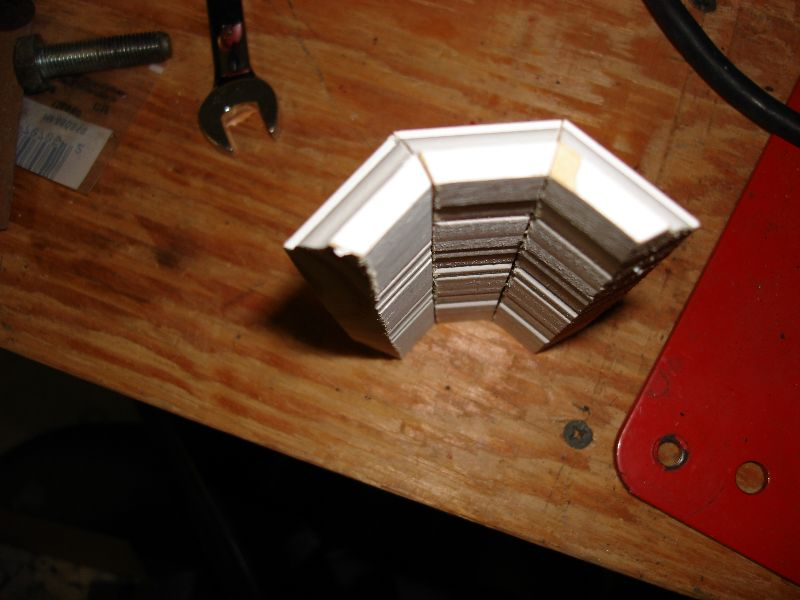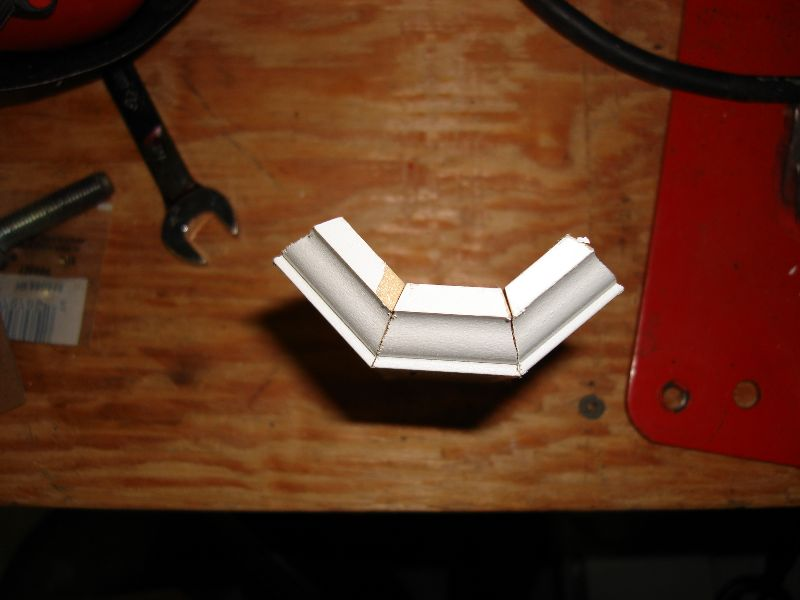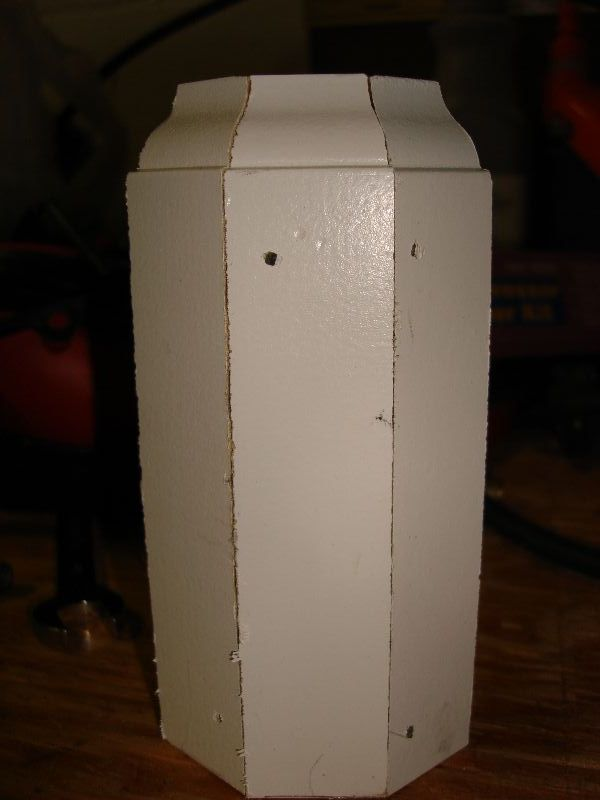### November 2007Converting Watts to Amps

The conversion of Watts to Amps is governed by the equation Amps = Watts/Volts

For example 12 watts/12 volts = 1 amp
Converting Amps to Watts

The conversion of Amps to Watts is governed by the equation Watts = Amps x Volts

For example 1 amp * 110 volts = 110 watts

Converting Watts to Volts

The conversion of Watts to Volts is governed by the equation Volts = Watts/Amps

For example 100 watts/10 amps = 10 volts

Converting Volts to Watts

The conversion of Volts to Watts is governed by the equation Watts = Amps x Volts

For example 1.5 amps * 12 volts = 18 watts

Converting Volts to Amps at fixed wattage

The conversion of Volts to Amps is governed by the equations Amps = Watts/Volts

For example 120 watts/110 volts = 1.09 amps

Converting Amps to Volts at fixed wattage

The conversion of Amps to Volts is governed by the equation Volts = Watts/Amps

For Example, 48 watts / 12 Amps = 4 Volts

high quality video here:
http://www.diuternity.com/vids/MOV01957.MPG

As you can see from the video, this printer bottoms out (scrapes the bottom of the ink carts)

Even it this printer would print correctly, how would it print if it bottoms out?

I love my bandsaw. Installing baseboards is easy! If you had only to cut 45degree angles only! I had to cut round corners which made the task a little challenging but I did it without a sweat! A whole house would probably take me 2 full days but now it’s only taking a day. To do round corner baseboards, you have to cut three pieces: three @ 22.5degrees!

Cutting the center piece that ties into the two boards is a pain! So I setup my bandsaw to cut the center 22.5degree piece. I needed twenty-four of them. Cutting them one at a time would prove to be very VERY time consuming. The bandsaw made it easy once I had setup a jig for it! Took me less than 30mins to cut all the pieces! Now all is needed are lots of other boards cut! I’ll finish tomorrow!

EDIT: Here are samples of the cuts I’m talking about: